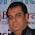## Pages

### Local Variable In Java Programming With Code Example and Sample

Video: ClickMe

#### LocalVariableInJava.java

```package com.variables.tutorialbyexample;

public class LocalVariableInJava {

public LocalVariableInJava() {
// TODO Auto-generated constructor stub
}

public static void print() {
int a = 50, b = 55, c = a + b;
System.out.println("Output of print() method variable a = " +
a + ", b = " + b + " and c =" + c);
}

public static void main(String[] args) {
// Local Variables
int a = 10;
int b = 20;
int c = a + b;
System.out.println("Output of main() method variable a = " +
a + ", b = " + b + " and c =" + c);

//Calling the static print method
print();

}
}
```

1.2.